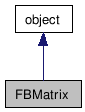# FBMatrix Class Reference

FBMatrix FBMatrix FBMatrix FBMatrix FBMatrix FBMatrix FBMatrix FBMatrix FBMatrix FBMatrix FBMatrix FBMatrix FBMatrix FBMatrix FBMatrix FBMatrix FBMatrix FBMatrix FBMatrix FBMatrix FBMatrix FBMatrix FBMatrix FBMatrix FBMatrix FBMatrix FBMatrix FBMatrix FBMatrix FBMatrix FBMatrix FBMatrix FBMatrix FBMatrix FBMatrix FBMatrix FBMatrix FBMatrix FBMatrix FBMatrix FBMatrix FBMatrix FBMatrix FBMatrix FBMatrix FBMatrix FBMatrix FBMatrix FBMatrix FBMatrix FBMatrix FBMatrix FBMatrix FBMatrix FBMatrix FBMatrix FBMatrix FBMatrix FBMatrix FBMatrix FBMatrix FBMatrix FBMatrix FBMatrix FBMatrix FBMatrix FBMatrix FBMatrix FBMatrix FBMatrix FBMatrix FBMatrix FBMatrix FBMatrix FBMatrix FBMatrix FBMatrix FBMatrix FBMatrix FBMatrix FBMatrix FBMatrix FBMatrix FBMatrix FBMatrix FBMatrix FBMatrix FBMatrix FBMatrix FBMatrix FBMatrix FBMatrix FBMatrix FBMatrix FBMatrix FBMatrix FBMatrix FBMatrix FBMatrix FBMatrix FBMatrix FBMatrix FBMatrix FBMatrix FBMatrix FBMatrix FBMatrix FBMatrix FBMatrix FBMatrix FBMatrix FBMatrix FBMatrix FBMatrix FBMatrix FBMatrix FBMatrix FBMatrix FBMatrix FBMatrix
Inheritance diagram for FBMatrix:[legend]

List of all members.

## Detailed Description

FBMatrix class.

Four x Four (double) Matrix.

This class creates a list like object, which can be modified using the list protocol method. But unlike lists, its length is fixed: it always contain 16 floating point values. Thus it does not support the any list methods that would affect its length. The values within can be changed, usually via the bracket operator.

```    # Supported list protocol methods:
mat = FBMatrix()
len(mat)
print mat
mat = 1.0
```
Warning:
The implementation of this 4x4 matrix uses a simple list of 16 elements, not a list of 4 vectors of 4 elements.*

Slicing is not supported by this object.

See sample: Matrix.py.

## Public Member Functions

FBMatrix (list pValue)
Constructor.
FBMatrix (FBMatrix pMatrix)
Copy Constructor.
Identity ()
Set (list pValue)
Set matrix from an array.
int  __len__ ()
Returns the number of elements.
float  __getitem__ (int pIndex)
Returns the ith component Corresponds to python: print matrix.
__setitem__ (int pIndex, float pComponentValue)
Sets the ith components Corresponds to python: color = 0.5.
FBMatrix ()
Constructor Initializes matrix to identity.
FBMatrix (list pValue)
Constructor.
FBMatrix (FBMatrix pMatrix)
Copy Constructor.

## Constructor & Destructor Documentation

 FBMatrix ( list pValue )

Constructor.

Parameters:
 pValue Array to intialize matrix from.

 FBMatrix ( FBMatrix pMatrix )

Copy Constructor.

Parameters:
 pMatrix Matrix to copy.

 FBMatrix ( )

Constructor Initializes matrix to identity.

 FBMatrix ( list pValue )

Constructor.

Parameters:
 pValue Array to intialize matrix from.

 FBMatrix ( FBMatrix pMatrix )

Copy Constructor.

Parameters:
 pMatrix Matrix to copy.

## Member Function Documentation

 Identity ( )

 Set ( list pValue )

Set matrix from an array.

Parameters:
 pValue Array to intialize matrix from.

 int __len__ ( )

Returns the number of elements.

Corresponds to python: len(object)

 float __getitem__ ( int pIndex )

Returns the ith component Corresponds to python: print matrix.

Parameters:
 pIndex Index of the components to get (0 to 15)
Returns:
Matrix element value

 __setitem__ ( int pIndex, float pComponentValue )

Sets the ith components Corresponds to python: color = 0.5.

Parameters:
 pIndex Index of the components to set (0 to 15) pComponentValue Value of component to set

Generated on Tue Feb 9 19:34:01 2010 for Python Reference Guide by1.5.3